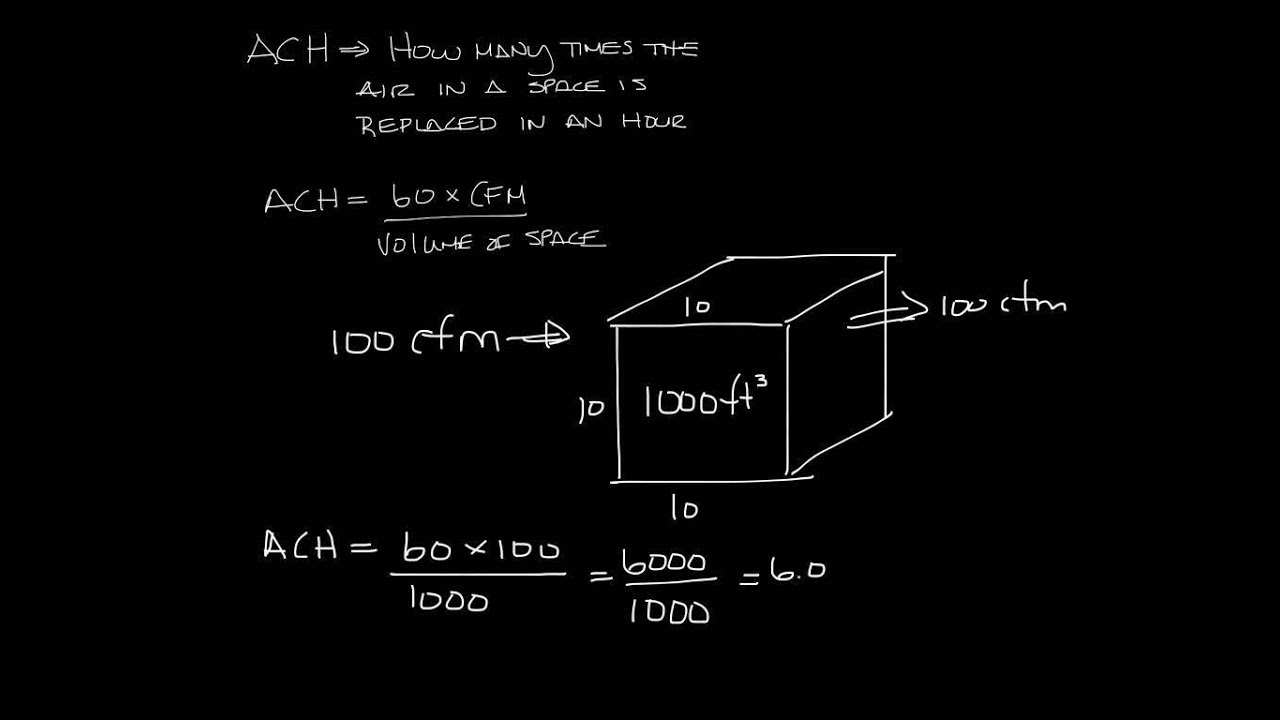# Simple Calculation For Air Changes Per HourAn HVAC system is usually dimensioned based on the heat load of the space that requires cooling. But the required ventilation needs, depending on both application and occupation, are often overlooked. A simple air change calculation may assist in finding the right amount of fresh air ventilation.

Simple calculation :-

• Use the table below to find the required amount of air changes per hour.
• Calculate the volume of the space to be conditioned in either m³ (for SI units) or in ft³ (for I-P units). Calculate the required airflow: Airflow

Air changes table :-
The table below shows the required air change rate values based on data from The Engineering Toolbox , Nuaire  and Technisch Adviesbureau Betuwe .